Related Articles

# Adding Straight Lines to a Plot in R Programming – abline() Function

• Last Updated : 14 Jul, 2020

`abline()` function in R Language is used to add one or more straight lines to a graph. The `abline()` function can be used to add vertical, horizontal or regression lines to plot.

Syntax:
abline(a=NULL, b=NULL, h=NULL, v=NULL, …)

Parameters:
a, b: It specifies the intercept and the slope of the line
h: specifies y-value for horizontal line(s)
v: specifies x-value(s) for vertical line(s)

Returns: a straight line in the plot

Example 1: To add a vertical line to the plot

 `# add line to square plot ``# first example : Add one line``plot``(cars)``abline``(v = 16, col = ``"darkgreen"``)`` ` `# second example : add 2 lines ``# addline to square plot ``# change line colors, sizes and types``plot``(cars)``abline``(v = ``c``(16, 22), col = ``c``(``"darkgreen"``, ``"blue"``),``                      ``lty = ``c``(1, 2), lwd = ``c``(1, 3))`` ` `# third example``set.seed``(1200); mydata<-``rnorm``(180)``hist``(mydata, col=``"darkgreen"``)`` ` `# lwd=line width, lty =linetype ``abline``(v = ``mean``(mydata), col = ``"blue"``, lwd = 4, lty = 4) `

Output: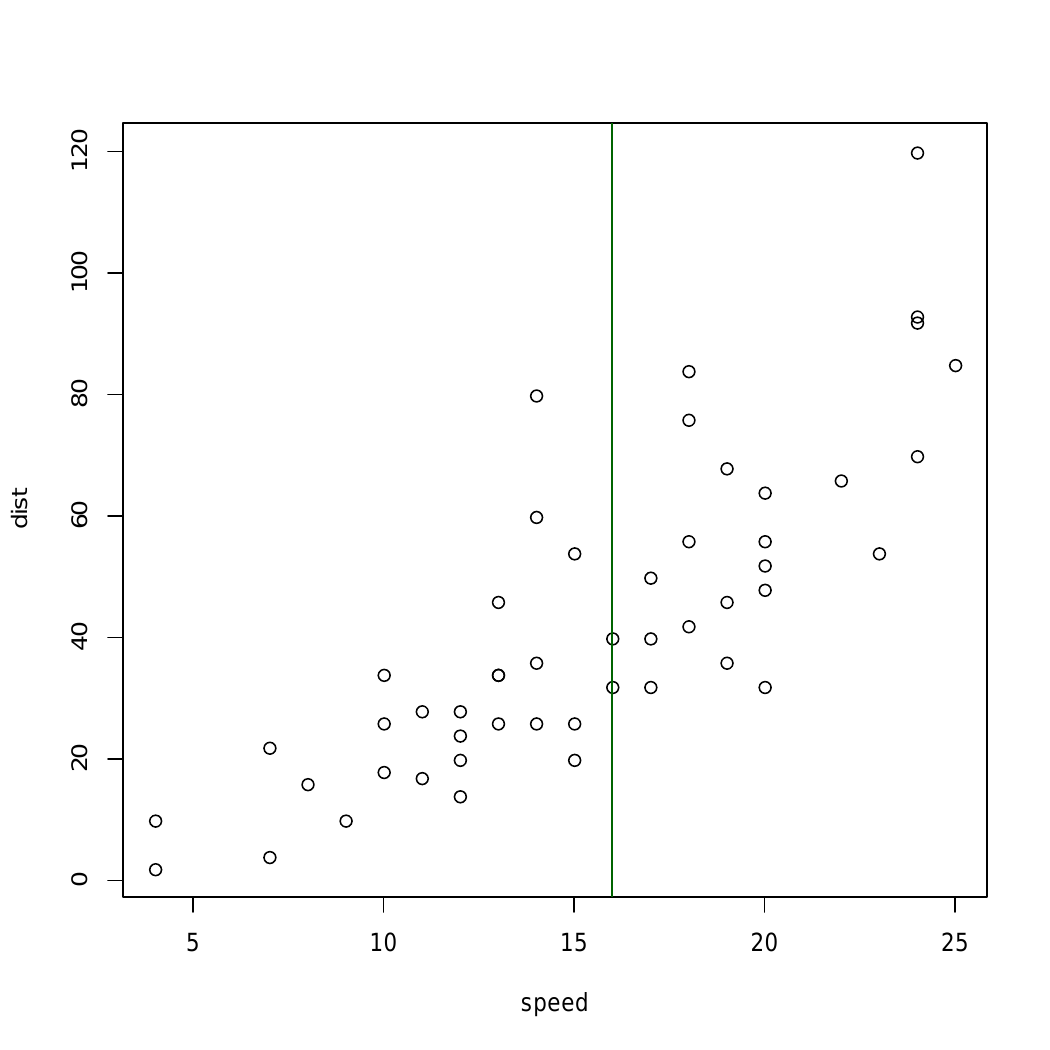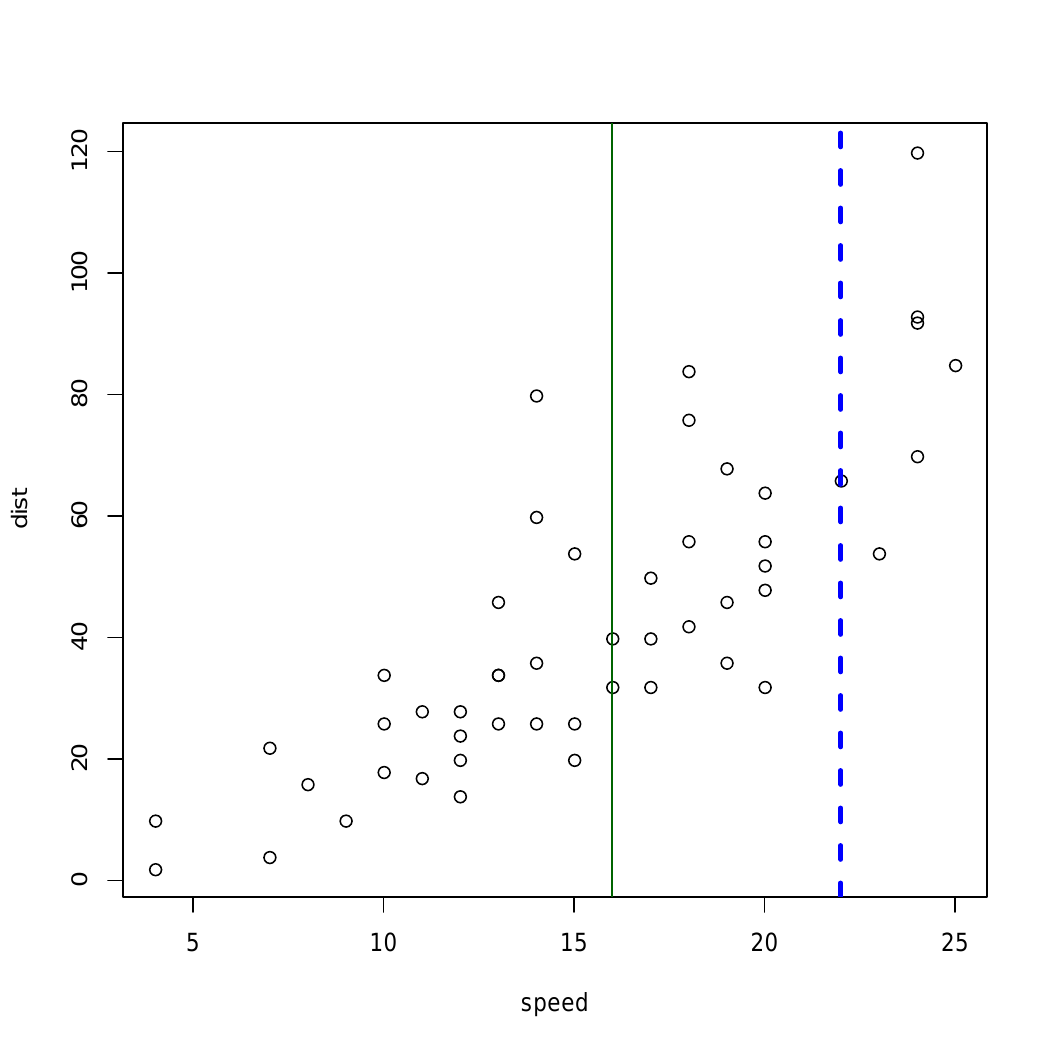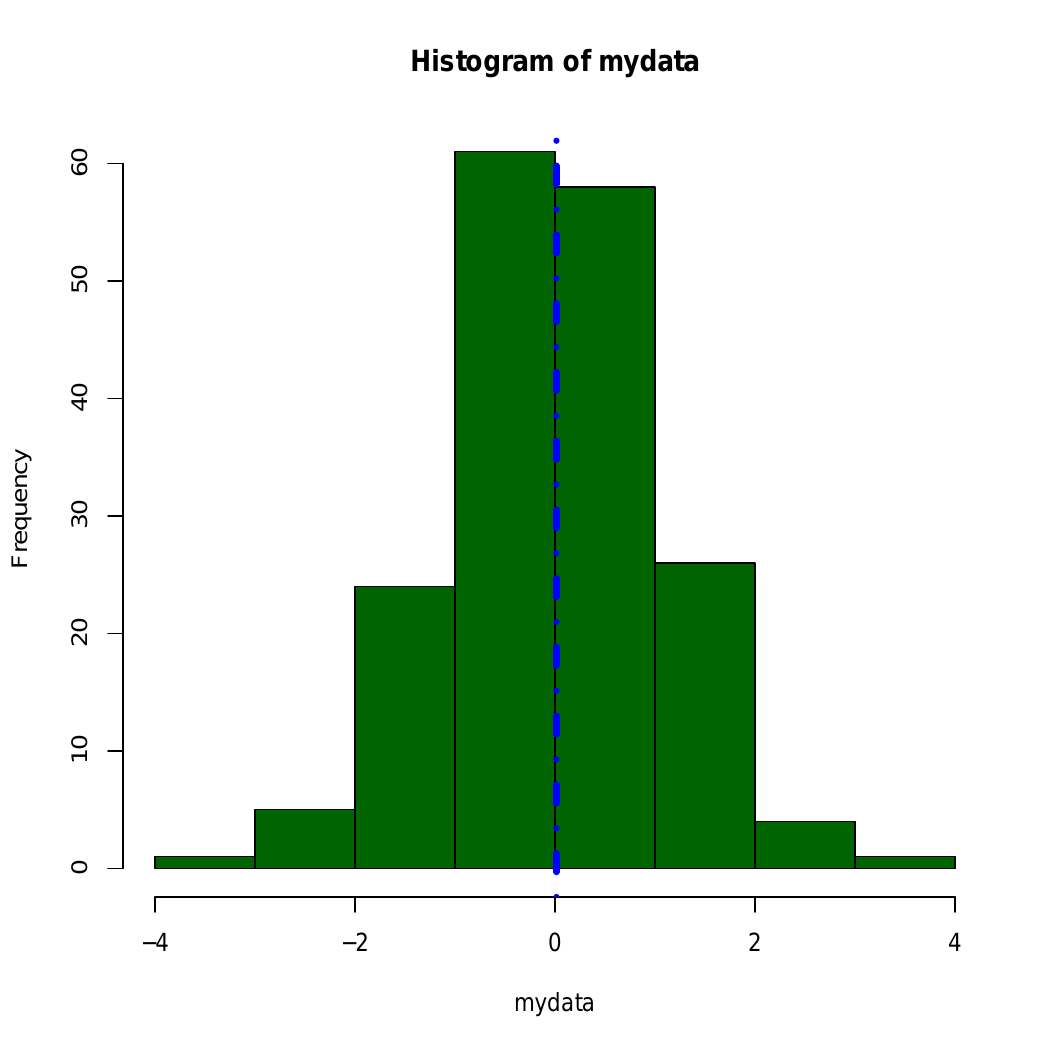Here, in above example straight line is added using abline() to different graphical plots

Example 2: To add a horizontal line

 `# R program to add a horizontal line``# to a plot`` ` `# Creating a plot``plot``(cars)`` ` `# Calling abline() function``abline``(h = 60, col = ``"darkgreen"``)`

Output: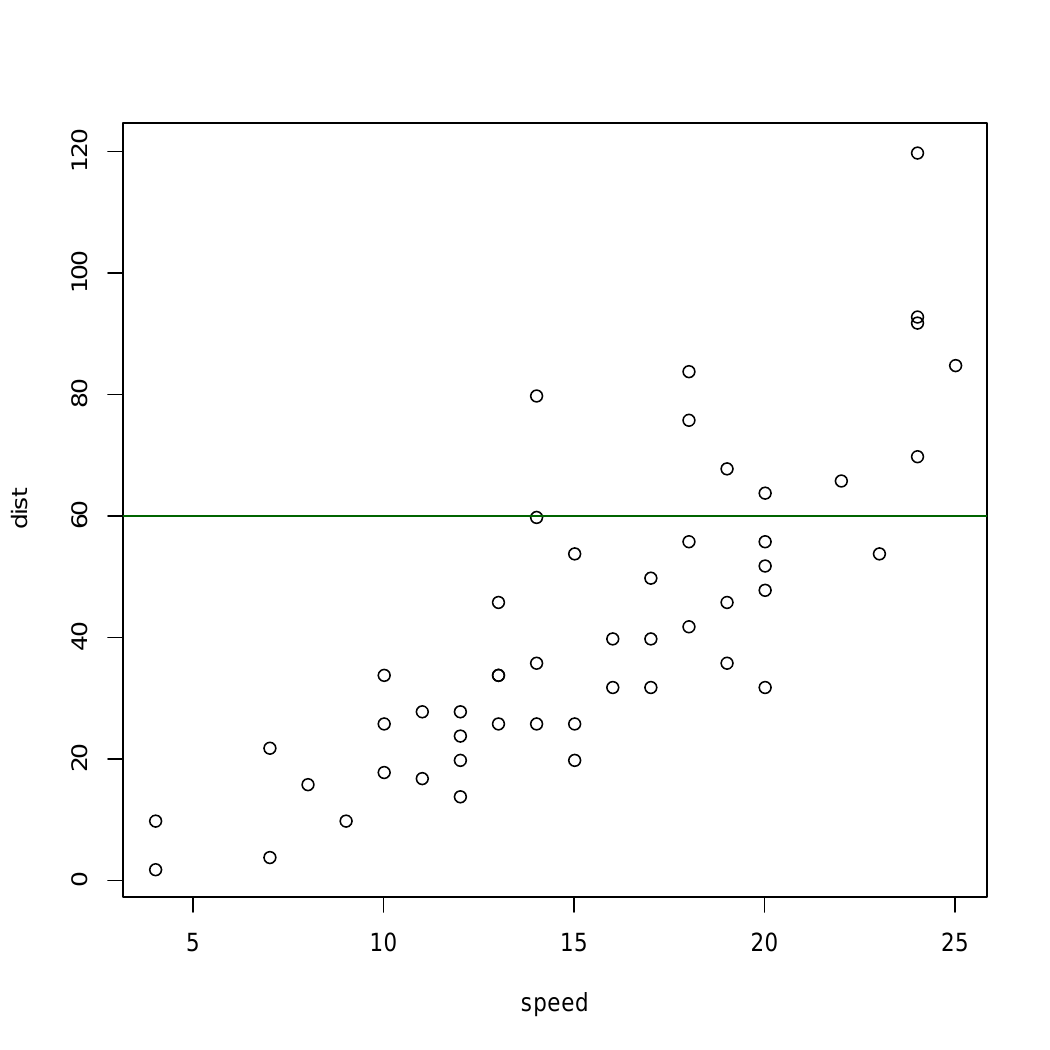In above example `abline()` Function draws an horizontal line on the current plot at the specified ‘x’ coordinates.

Example 3: To add a regression line

 `par``(mgp = ``c``(2, 1, 0), mar = ``c``(3, 3, 1, 1))`` ` `# Fit regression line``require``(stats)``reg<-``lm``(dist ~ speed, data = cars)``coeff = ``coefficients``(reg)`` ` `# equation of the line : ``eq = ``paste0``(``"y = "``, ``round``(coeff, 1), ``"*x "``, ``                    ``round``(coeff, 1))`` ` `# plot``plot``(cars, main = eq)``abline``(reg, col = ``"darkgreen"``)`

Output: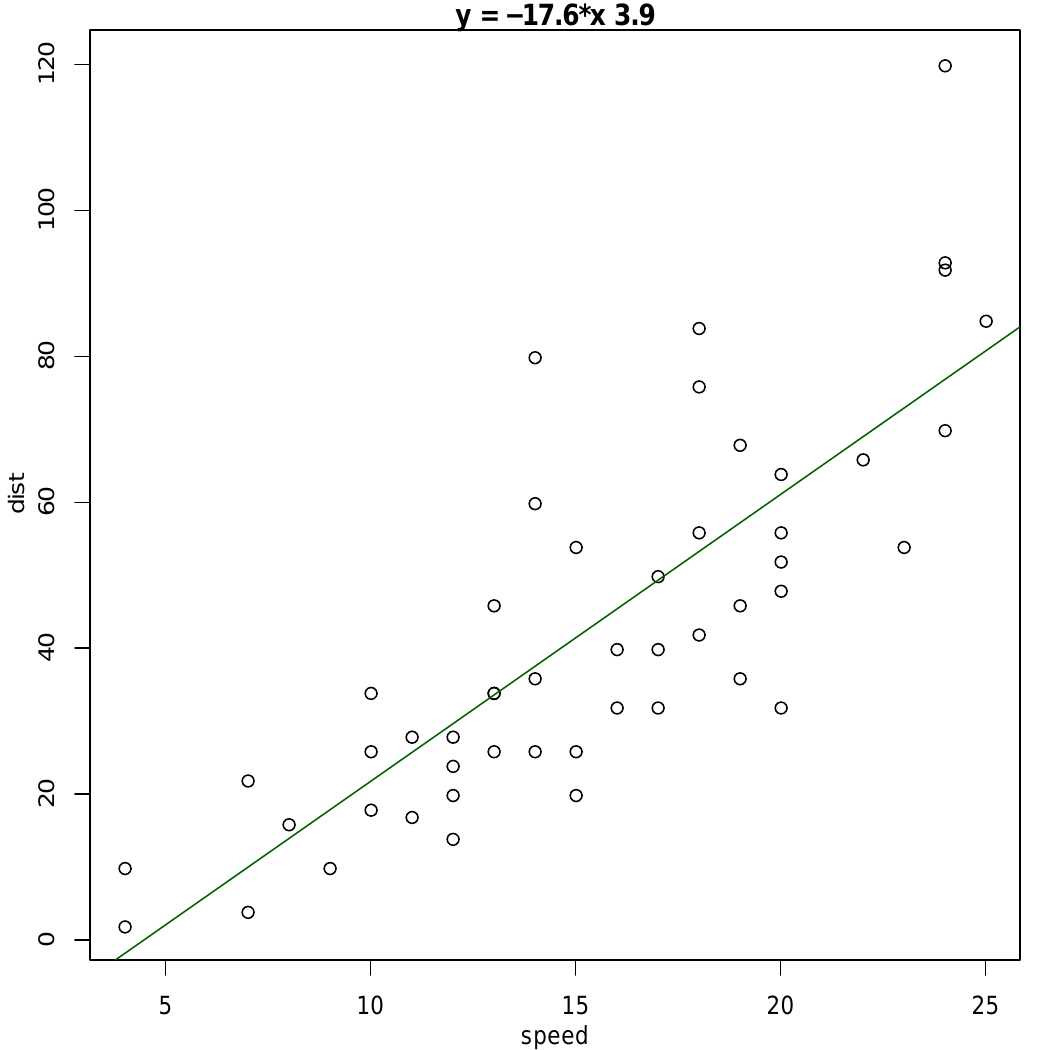In the above example, straight-line is added using the line equation and `abline()` function and plot relation between speed and distance.

My Personal Notes arrow_drop_up